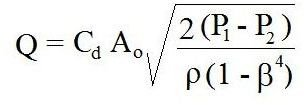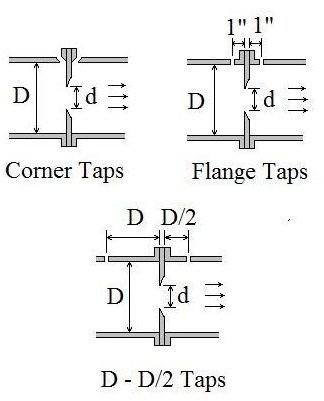# Orifice Flow Meter Discharge Coefficient Values Using ISO 5167

Page content

## Introduction

ISO 5167, which came out in 1991, set three standard configurations for the pressure taps in an orifice flow meter. It also provided means to calculate the orifice discharge coefficient for any ratio of orifice diameter to pipe diameter if one of the standard pressure tap configurations is used. Thus ISO 5167 allows an orifice flow meter to do fluid flow measurement over a wide range of flow rate because the orifice plates in a given orifice flow meter can be changed while still allowing accurate determination of the orifice discharge coefficient.

## Orifice Flow Meter Background

The orifice meter is the simplest type of differential pressure flow measurement device. It is just a circular plate (the orifice plate) with a hole in themiddle, usually held in place between pipe flanges as shown in the figure at the right. The flow is accelerated due to the constriction, so the pressure is decreased downstream of the orifice plate. The pressure difference, P1 - P2, as shown in the figure, can be measured and used to calculate the flow rate passing through the meter from the equation at the right. The equation gives flow rate, Q, in terms of the measured pressure difference, P1 - P2, the density of the fluid, ρ, the ratio of orifice diameter to pipe diameter, β, the cross-sectional area of the orifice, Ao, and the orifice discharge coefficient, Cd.

For more details about the orifice, flow nozzle, and venturi meter, see the article, “The Orifice, Flow Nozzle, and Venturi Meter for Pipe Flow Measurement.”

## Pressure Tap Locations

The typical locations of the pressure taps for an orifice flow meter have changed over time. Prior to 1991, the downstream pressure tap was typically located at the vena contracta, which is the minimum jet area, downstream from the orifice plate, as shown in the figure in the previous section. The correlations for determination of the orifice discharge coefficient, Cd, were based on a vena contracta downstream pressure tap. The downstream distance of the vena contracta from the orifice plate, however changes with different orifice diameters, so changing orifice sizein a given orifice flow meter (to change the range of flow rate measurement) required moving the downstream pressure tap in order to get an accurate theoretical estimate of the orifice discharge coefficient.

In 1991 ISO 5167 identified three standard pressure tap configurations for an orifice flow meter. The three configurations are known as corner taps, flange taps, and D - D/2 taps, as illustrated in the figure at the left. The advantage of the ISO 5167 pressure tap configurations is that the distance of the pressure taps from the orifice plate is given as a fixed distance, or as a function of the pipe diameter, independent of the orifice diameter.

## Determination of Orifice Discharge Coefficient

ISO 5167 provides an equation (given below) for the orifice discharge coefficient, Cd, as a function of β (d/D), Reynolds number (Re), L1 and L2, where L1 is the distance of the upstream pressure tap from the orifice plate and L2 is the distance of the downstream pressure tap from the orifice plate. As shown in the figure above L1 = L2 = 0 for corner taps; L1 = L2 = 1" for flange taps; and L1 = D & L2 = D/2 for D-D/2 taps. The equation for the orifice discharge coefficient is:

Cd - 0.5959 + 0.0312 β2.1 - 0.1840 β8 + 0.0029 β2.5(106/Re)0.75 + 0.0900(L1/D)[β4/(1 - β4)] - 0.0337(L2/D)β3

This equation can be used to find the orifice discharge coefficient for an orifice flow meter with any of the three standard pressure tap configurations, but not for any other arbitrary values of L1 and L2. The introduction of standard pressure tap configurations and the equation for Cd, allows a given orifice flow meter to conveniently use different size orifice openings and cover a wide flow measurement range.

Note that an iterative (trial and error) calculation is needed to get a value for Cd, because the upstream velocity needed for Re isn’t known until Cd is known. An Excel spreadsheet works well for the iterative calculation of the orifice discharge coefficient using this equation. For a downloadable Excel template for the use of this equation, see the article, “Excel Templates for Venturi and Orifice Flow Meter Calculations.”

A U.S Dept. of the Interior reference with information about ISO 5167 for determining the orifice discharge coefficient is provided below.

## Images and Reference

Images drawn by H. Bengtson

REFERENCES for further information:

1. U.S. Dept. of the Interior, Bureau of Reclamation, 2001 revised, 1997 third edition, Water Measurement Manual.

2. International Organization of Standards - ISO 5167-1:2003 Measurement of fluid flow by means of pressure differential devices, Part 1: Orifice plates, nozzles, and Venturi tubes inserted in circular cross-section conduits running full. Reference number: ISO 5167-1:2003.

3. Bengtson, Harlan H., Flow Measurement in Pipes and Ducts__, An online continuing education course.

## This post is part of the series: Measurement of Pipe Flow Rate

Measurement of pipe flow rate can use various flow meters, including a differential pressure flowmeter, like the orifice meter, venturi meter and flow nozzle meter. Other types of liquid flow meter are the rotameter, magnetic flow meter, ultrasonic meter, turbine flow meter and coriolis flow meter.